# Short Tricks to solve Maths Questions in Management Exams

Author : Palak Khanna

Updated On : November 24, 2023

SHARE

Summary: Unlock the secrets of maths to ace the Quantitative Aptitude section in the IPMAT exam. Master the art of cross-multiplication and solve complex problems in seconds. Elevate your exam strategy now!

Are you preparing for the IPMAT exam but struggling to manage time while solving the Quantitative Aptitude section? Well, some simple techniques of vedic maths can help you solve complex calculations and equations in a matter of seconds.

Are you wondering how? Read through the post below to get a thorough understanding of cross multiplication, as well as its implementation by IPM Aptitude Questions and Answers.

## Maths Short Tricks for Management Entrances After 12th

The QA section in management entrances is a bit difficult as can be seen from the cut-off trends. Mathematics questions are lengthy and time-consuming, therefore it is advised to follow important tips and tricks of vedic maths to maximise your attempts.

One of the important concepts to save time in multiplication is the cross-multiplication method based on Vedic maths. Cross Multiplication will help you solve equations in seconds which would help you save time for other questions.

Check: IPMAT 2024 Exam Dates & Schedule

### What is cross multiplication?

In solving linear equations with two variables, the cross multiplication method is used. The method of cross-multiplication is the simplest and most straightforward method of solving linear equations in two variables. When we have a pair of variables in a linear equation, we use this method the most.

Cross-multiplication is a technique to determine the solution of linear equations in two variables. It proves to be the fastest method to solve a pair of linear equations. For a given pair of linear equations in two variables.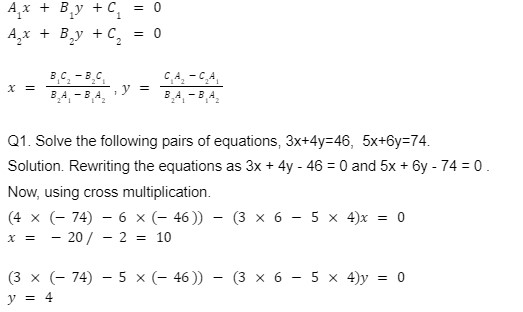Prepare with:  IPMAT Mock Test 2024

How to use cross multiplication to solve questions in management entrance exams?

This trick can be used in multiple types of questions like average based, profit and loss, mixture and allegations or time and work. Lets understand this trick using an example.

Q1. A person sold an article for 8(1/3)% profit. Had he bought the article at Rs. 120 less and sold it for Rs. 180 less, there would be a loss of 33(1/3)%, find the initial C.P. of the article.

Solution.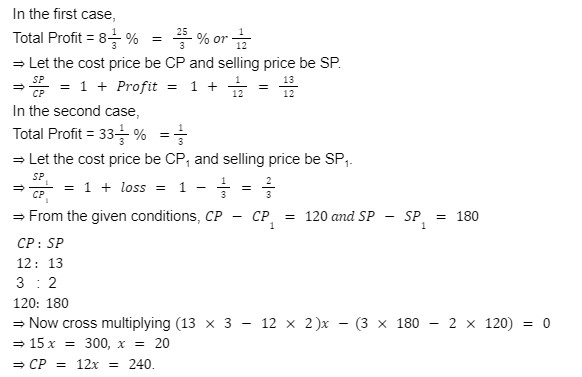Practice: Quantitative Aptitude Questions on Heights and Distance for IPMAT & DU JATQ2. In an exam, the ratio of selected to unselected candidates was 6:1. If 30 less had applied and 10 less selected, the ratio of selected to unselected would have been 7:1. How many candidates had applied for the exam?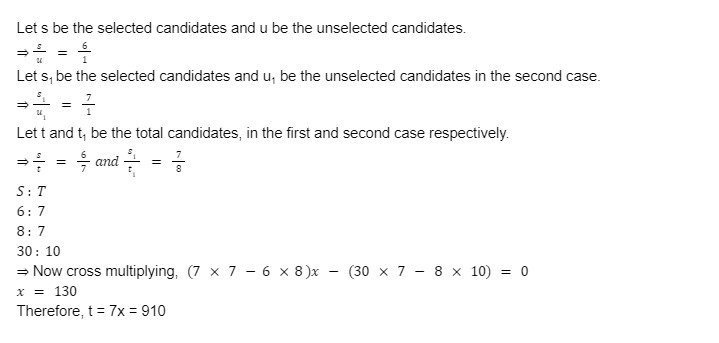Q3. A and B earn in the ratio 2: 1. They spend in the ratio 5:3 and save in the ratio of 4: 1. Find the monthly income of each if the total monthly savings of both A and B together is Rs. 5000 ?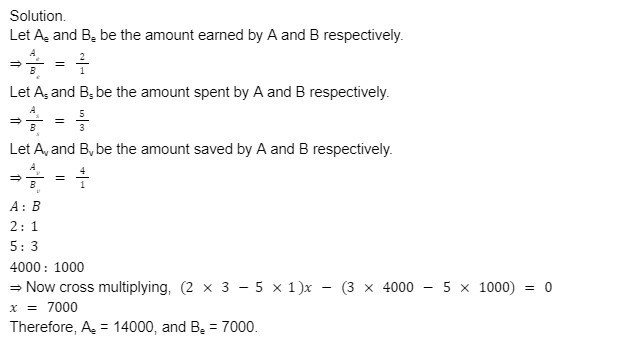Q4. If salary of A is Rs. 300 more than that of B. If Salary of A is increased by 16.66% and that of B is increased by 14(2/7)%, A's salary would be Rs. 400 more than B. Find A's salary.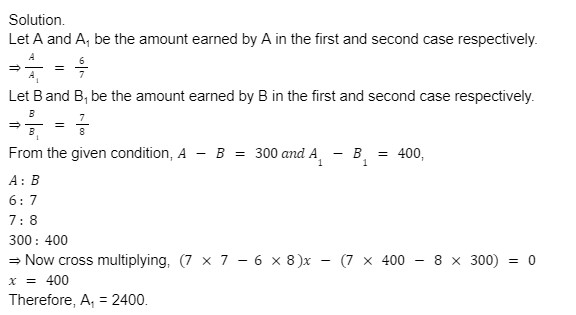Check: IPM Aptitude Trailing Zeroes Questions & Answers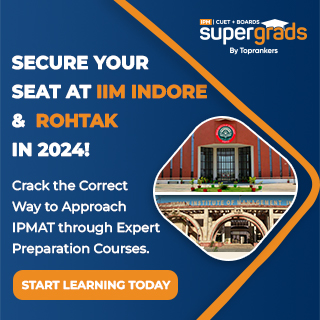Q5.  A vessel contains a mixture of milk and water in the ratio of 14 : 3. Now, 25.5 litres of the mixture is taken out from the vessel and 2.5 litres of pure water and 5 litres of pure milk is added to the mixture. If the resultant mixture contains 20% water, what was the initial quantity of mixture in the vessel before the replacement? (in litres).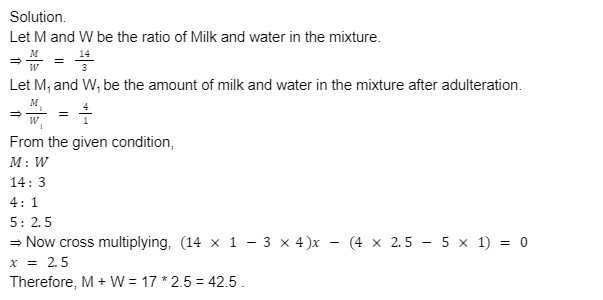In conclusion, the utilization of Vedic maths, particularly the cross-multiplication method, offers a significant advantage for IPMAT aspirants tackling the Quantitative Aptitude section. This approach not only simplifies complex calculations but also significantly reduces the time required to solve them.

By incorporating these techniques into their study plan, candidates can enhance their problem-solving speed and accuracy, giving them a competitive edge in the exam. This article serves as a valuable resource for students seeking efficient strategies to excel in competitive management entrance exams.

Read more: IPMAT Study Plan 2024

What is the best strategy to manage time efficiently in the maths section of Management exams?How to excel in management entrance exams like IPMAT and DUJAT?Should I join a coaching class to clear management entrance exams?How to prepare for management entrance exams while preparing for boards?How to create the best study plan?How to prepare for the Quantitative aptitude section of Management entrance exams?